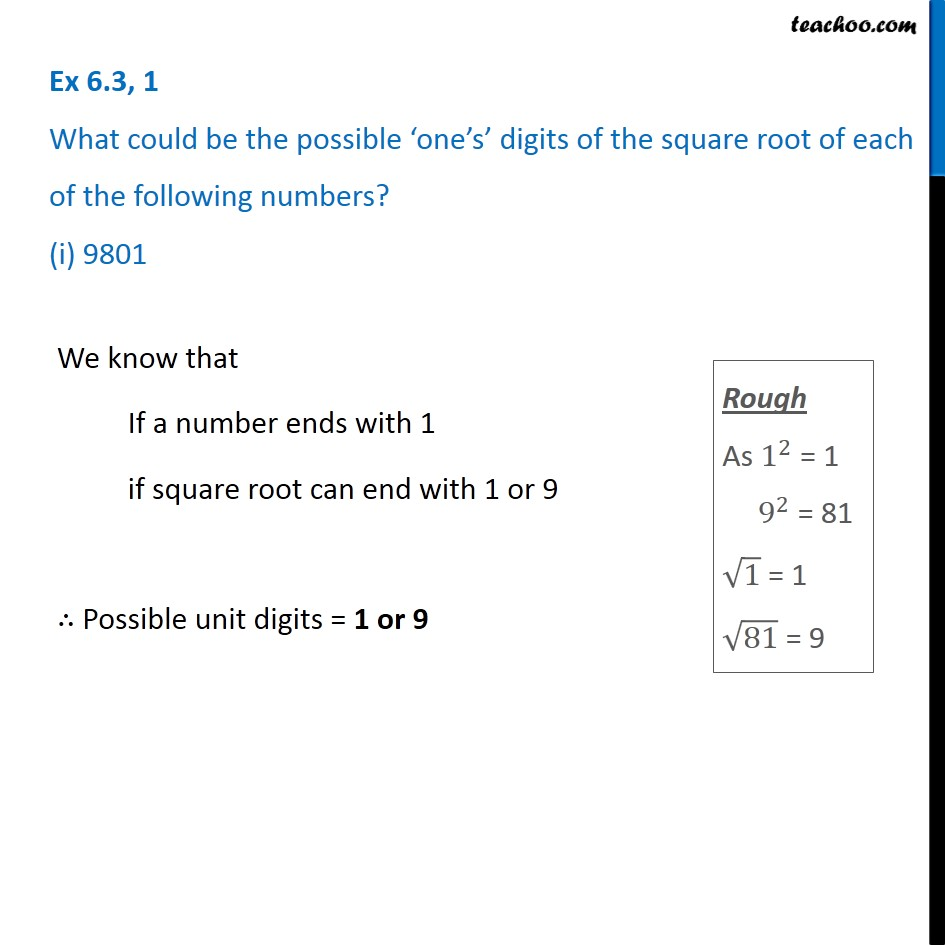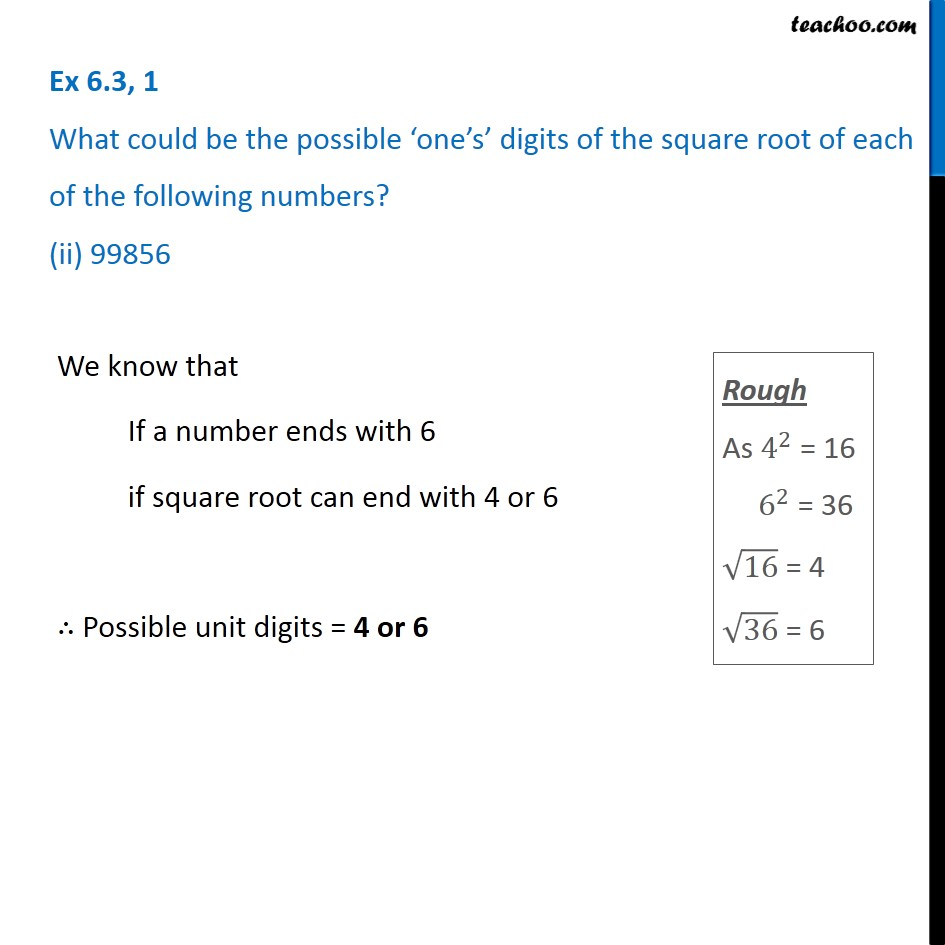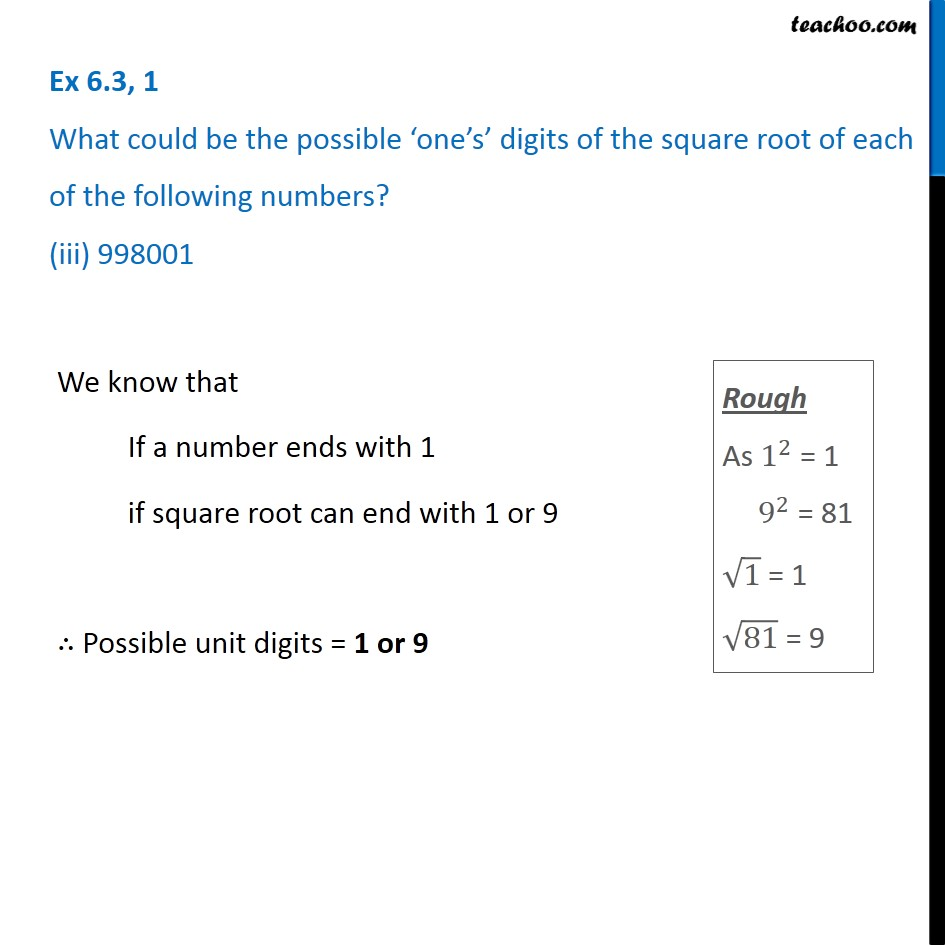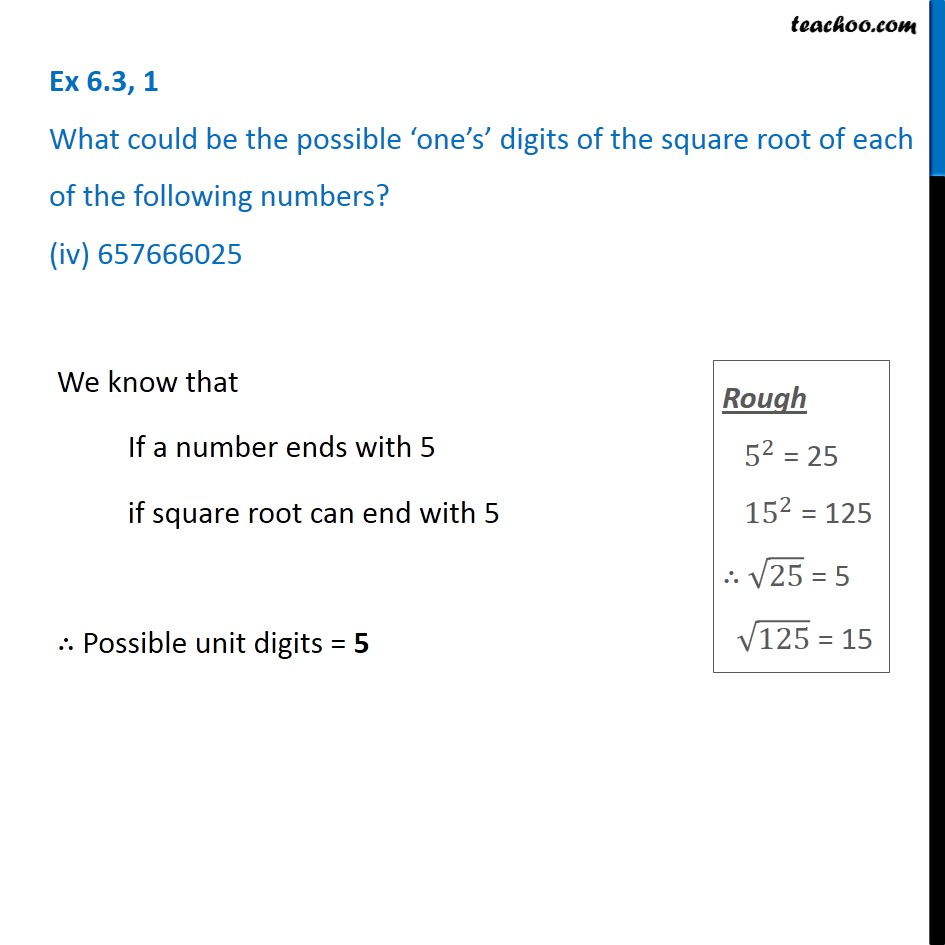Subscribe to our Youtube Channel - https://you.tube/teachoo

1. Chapter 6 Class 8 Squares and Square Roots
2. Concept wise
3. Square root

Transcript

Ex 6.3, 1 What could be the possible ‘one’s’ digits of the square root of each of the following numbers? (i) 9801We know that If a number ends with 1 if square root can end with 1 or 9 ∴ Possible unit digits = 1 or 9 Ex 6.3, 1 What could be the possible ‘one’s’ digits of the square root of each of the following numbers? (ii) 99856We know that If a number ends with 6 if square root can end with 4 or 6 ∴ Possible unit digits = 4 or 6 Ex 6.3, 1 What could be the possible ‘one’s’ digits of the square root of each of the following numbers? (iii) 998001We know that If a number ends with 1 if square root can end with 1 or 9 ∴ Possible unit digits = 1 or 9 Ex 6.3, 1 What could be the possible ‘one’s’ digits of the square root of each of the following numbers? (iv) 657666025We know that If a number ends with 5 if square root can end with 5 ∴ Possible unit digits = 5

Square root

About the AuthorDavneet Singh
Davneet Singh is a graduate from Indian Institute of Technology, Kanpur. He has been teaching from the past 9 years. He provides courses for Maths and Science at Teachoo.